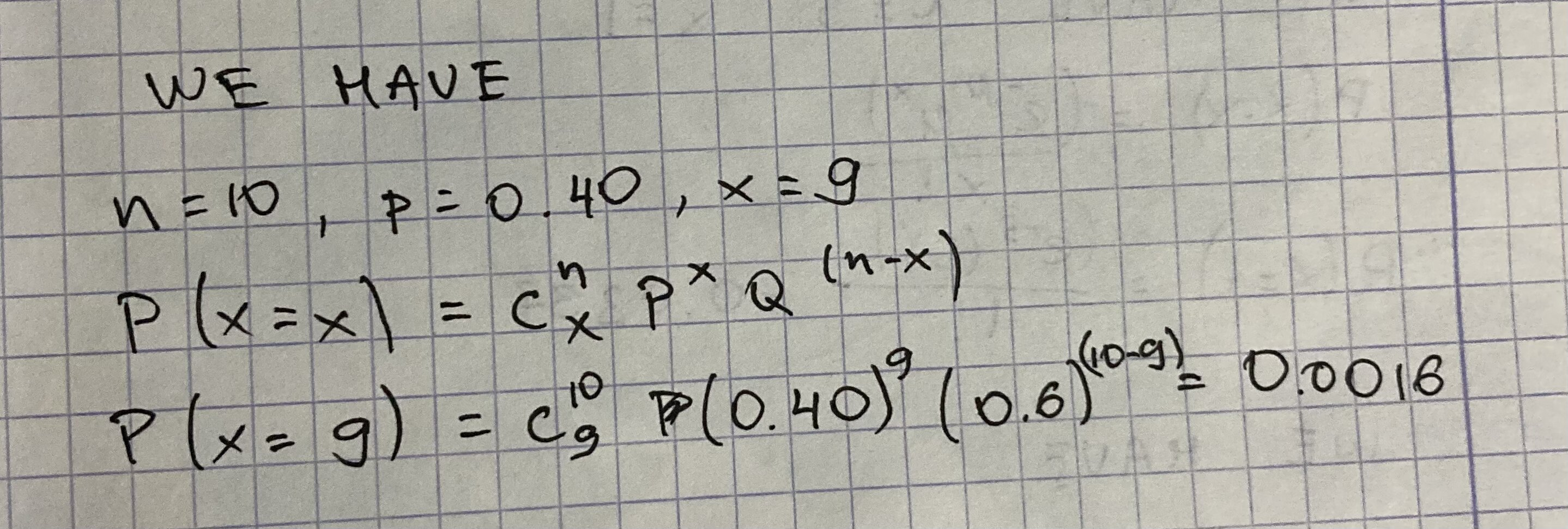# Question# Solve the binomial probability when n=10, p=0.40, x=9

Binomial probability
ANSWEREDSolve the binomial probability when n=10, p=0.40, x=9